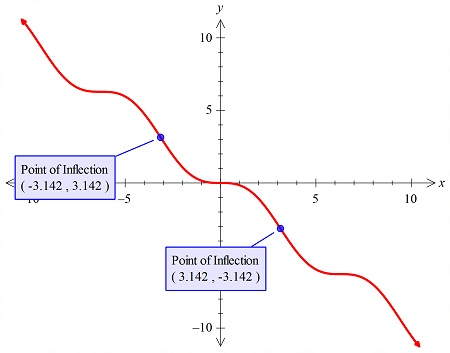# Find local max and min (if any), inflection points (if any), of f(x) = sin(x) - x.

## Question:

Find local max and min (if any), inflection points (if any), of {eq}f(x) = \sin(x) - x {/eq}.

## Graphs:

The graph of the function of type: {eq}f(x) = a\sin(x) - b x {/eq}

can have at least two inflection points, as these graphs are symmetric about the origin. And this graph will not have any maxima or the point.

## Answer and Explanation:

The graph of the function:

{eq}f(x) = \sin(x) - x {/eq}.

is plotted below:we see that there is two inflection point, they are at: {eq}x = \pm \pi {/eq}

#### Learn more about this topic:Graphs: Types, Examples & Functions

from High School Algebra II: Help and Review

Chapter 16 / Lesson 11
355K